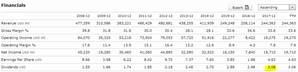Chapter 9, Problem 6TCLFundamentals of Financial Manageme...

15th Edition
Eugene F. Brigham + 1 other
ISBN: 9781337395250

Solutions

Chapter
SectionFundamentals of Financial Manageme...

15th Edition
Eugene F. Brigham + 1 other
ISBN: 9781337395250
Textbook Problem

Estimating Exxon Mobil Corporation's Intrinsic Stock ValueUse online resources to work on this chapter's questions. Please note that website information changes over time, and these changes may limit your ability to answer some of these questions.In this chapter, we described the various factors that influence stock prices and the approaches that analysts use to estimate a stock’s intrinsic value. By comparing these intrinsic value estimates to the current price, an investor can assess whether it makes sense to buy or sell a particular stock. Stocks trading at a price far below their estimated intrinsic values may be good candidates for purchase, whereas stocks trading at prices far in excess of their intrinsic value may be good stocks to avoid or sell Although estimating a stock's intrinsic value is a complex exercise that requires reliable data and good judgment, we can use the Internet to find financial data in order to arrive at a quick "back-of-the- envelope" calculation of intrinsic value.6. In the text, we discussed using the discounted dividend model to estimate a stock's intrinsic value. To keep things as simple as possible, let's assume at first that XOM’s dividend is expected to grow at a constant rate of 5% annually over time. So, g = 5%. If so, the intrinsic value equals Dt/(rs – g), where D1, is the expected annual dividend 1 year from now, r is the stock’s required rate of return, and g is the dividend’s constant growth rate. Go back to the summary (overview) screen and find XOM's current dividend. Multiply this dividend by 1 + g to arrive at an estimate of D1.Summary Introduction

To calculate: The amount of next year dividend per share.

Introduction:

Dividends:

It refers to the return over shares that is provided to the shareholders as a part of their earnings on the shares that they have invested in a company. It is that part of the total earnings that the company distributes among its shareholders.

Explanation

Given information:

Dividend per share of the company provided current year is \$3.06 per share. Growth rate of dividend per share of the company is 5%.

The formula to calculate the dividend per share for next year is,

D1=D0×(1+g)

Where,

• D1 is the dividend per share for the next year.
• D0 is the dividend per share for the current year

Still sussing out bartleby?

Check out a sample textbook solution.

See a sample solution

The Solution to Your Study Problems

Bartleby provides explanations to thousands of textbook problems written by our experts, many with advanced degrees!

Get Started

What does the invisible hand of the marketplace do?

Brief Principles of Macroeconomics (MindTap Course List)

Why do economists make assumptions?

Principles of Economics (MindTap Course List)

CAPM AND REQUIRED RETURN HR Industries (HRI) has a beta of 1.8, while LR Industries (LRI) beta is 0.6. The risk...

Fundamentals of Financial Management, Concise Edition (with Thomson ONE - Business School Edition, 1 term (6 months) Printed Access Card) (MindTap Course List)